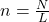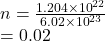## How many atoms are in 1.204×10^22 moles of arsenic?

Question

How many atoms are in 1.204×10^22 moles of arsenic?

in progress 0
6 months 2021-08-22T05:41:17+00:00 1 Answers 3 views 0

## 0.02 moles

Explanation:

To find the number of moles in a substance given it’s number of entities we use the formulawhere n is the number of moles

N is the number of entities

L is the Avogadro’s constant which is

6.02 × 10²³ entities

We haveWe have the final answer as

### 0.02 moles

Hope this helps you